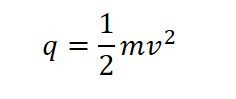Search
•Shalmali

# What is Dynamic Pressure in Rockets?

Updated: May 4, 2021

What is "Max-Q"?

Have you seen a live launch of a space shuttle? It looks beautiful, isn’t it? Have you ever wondered what is “Max-Q”, as they announce the Max-Q of the vehicle during the launch?

Get along to understand what Max-Q is! Before actually getting into Dynamic Pressure we need to understand a few basic terminologies, let's get into it first!

# Dynamic Pressure:

### Forces acting on the rocket:

What are the forces acting on the rocket? Seems very difficult to even guess right? Well, let's simplify it!

There are five types of forces acting on the rocket, as follows:

1. Gravitational force is the force acting on the rocket due to the gravity of the earth. (Well! no one can escape the gravitational force.)

2. Pressure force is the force acting on the rocket due to the presence of air around the rocket.

3. Viscous force is the force acting on the rocket due to the viscosity of the air.

4. Compressible force is the force acting on the rocket due to the property of compressibility of air.

5. Turbulent force is the force acting on the rocket due to the turbulence caused in the air especially at the end of the rocket.

Considering all these forces at once seems complicated, isn't it? To make it simple, let's neglect Compressible and Turbulent force. Naiver-Stokes equation consists of Gravitational, Pressure, and Viscous Force, after neglecting Compressible and Turbulent force.

But still seems difficult, isn't it? Again, to further simplify it, we ignore the Viscous force too. This equation is called Euler’s Equation. (Euler's Equation consists of Gravitational and Pressure Force). When we integrate Euler’s Equation, we get Bernoulli’s Equation. This Bernoulli’s Equation is very important to understand Dynamic Pressure.

It basically goes like this:

• Gravitational, Pressure, Viscous, Compressible & Turbulent Forces:- Act on Rocket

• Gravitational, Pressure & Viscous Forces:- Naiver-Stokes Equation

• Gravitational & Pressure Forces:- Euler’s Equation, after integration Bernoulli's Equation

### Bernoulli's Equation:

We consider only two forces (Gravitational Force and Pressure Force) in Bernoulli’s Equation. Bernoulli’s Equation is derived from the law of conservation of energy, which implies as: The energy of the flowing fluid at any point in the fluid is constant.

In the above equation, the first term (P1 and P2) is the Pressure Force and the last term is Gravitational Force. Then what is the middle term? This middle term is Dynamic Pressure. From the middle term, we can interpret that when a fluid is moving or it has some velocity, then the Dynamic Pressure will exist.

Dynamic Pressure exists only when the fluid is in motion or it has some velocity.Dynamic Pressure

Dynamic Pressure is denoted by "q", with units Pascal or N/sq.m. The maximum Dynamic Pressure acting on the rocket during the launch is called “Max-Q”.

### Summary:Dynamic Pressure
1. Gravitational, Pressure, Viscous, Compressible & Turbulent Forces act on Rocket.

2. Neglecting other Forces, we derive Bernoulli's Equation which takes care of Gravitational and Pressure Forces.

3. Dynamic Pressure exists only when the fluid has velocity.

4. The maximum Dynamic Pressure acting on the rocket during the launch is called “Max-Q”.

If this is Dynamic Pressure, then what is Static Pressure? Want to know more about the topic in detail? Follow me for similar topics!